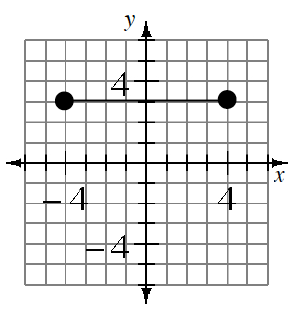### Home > ACC6 > Chapter 3 Unit 3 > Lesson CC1: 3.2.4 > Problem3-129

3-129.

Name the endpoints of the segment shown on the graph at right. What is the length of the segment? Write an absolute value expression to show how to calculate the length of the segment.

The segment is horizontal with one endpoint $4$ units to the left of the $y$-axis and one endpoint that is $4$ units to the right of the $y$-axis. You can use the expression below to determine the length of this segment.

$\left|−4\right| + \left|4\right|$